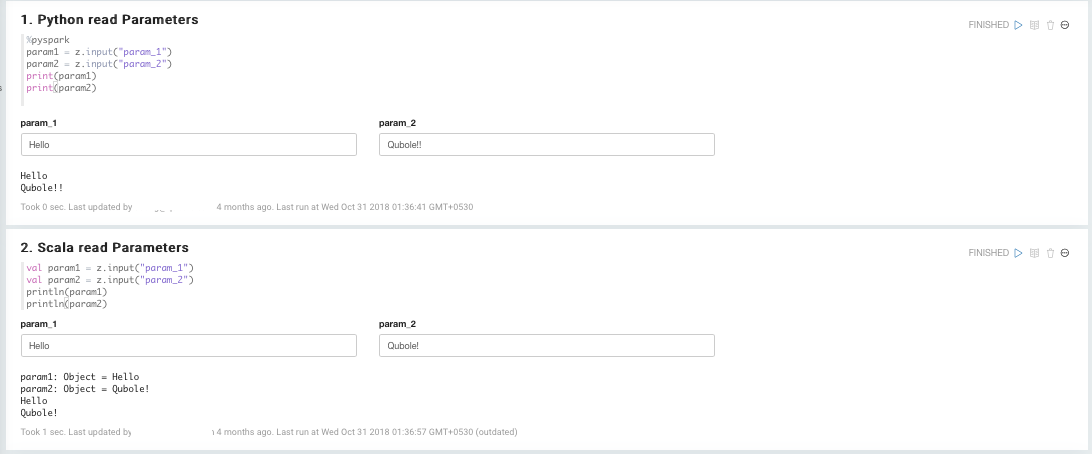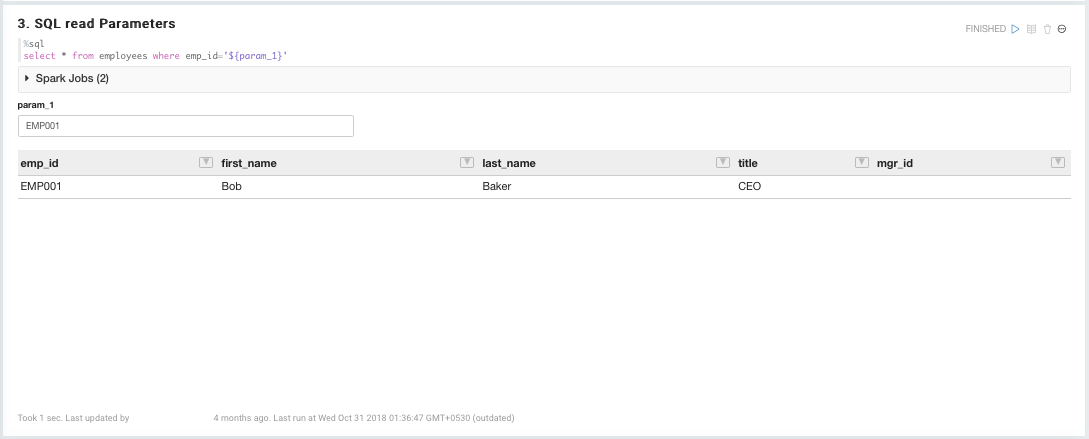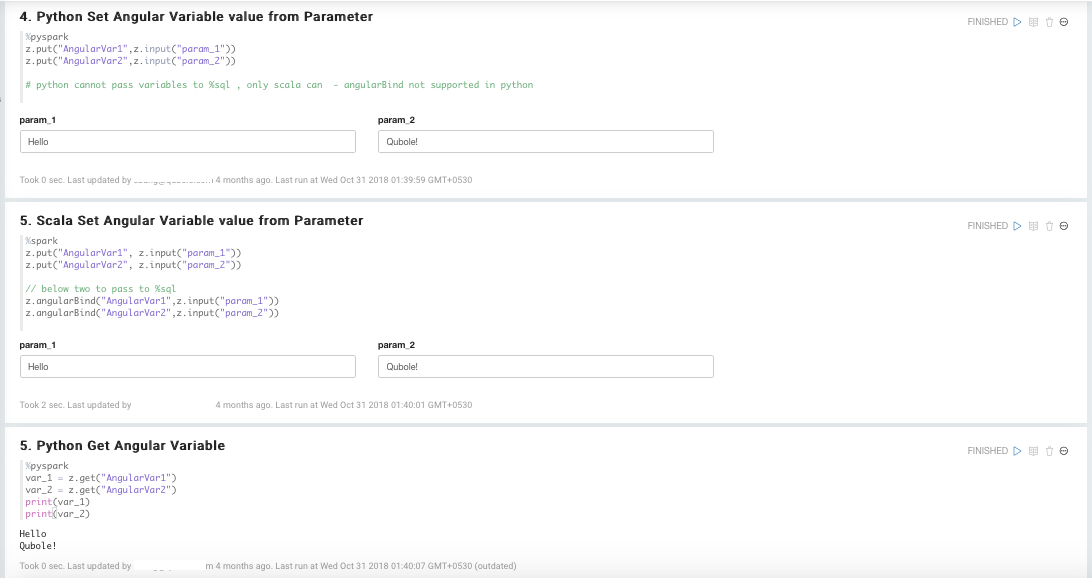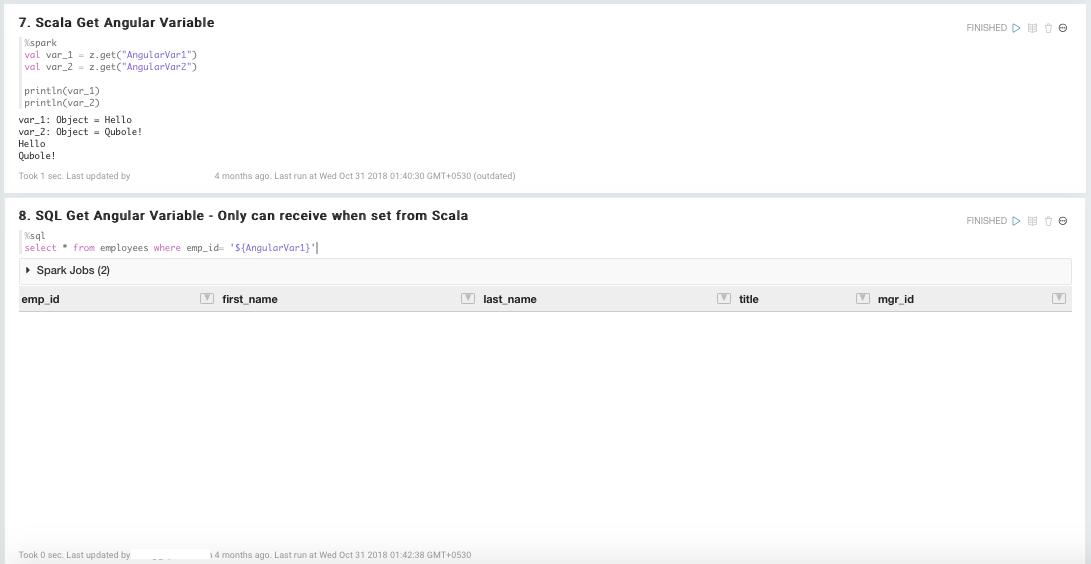# Parameterizing Notebooks¶

If you want to run notebook paragraphs with different values, you can parameterize the notebook and then pass the values from the Analyze or Scheduler page in the QDS UI, or via the REST API.

## Defining Parameters¶

You should define the parameters that have to be passed to the cell when running the notebook through various interfaces.

The following examples show how to define parameters.

### Python, Scala, and SQL¶

The following example shows how to define Python read parameters.

```%pyspark
param1 = z.input("param_1")
param2 = z.input("param_2")
print(param1)
print(param2)
```

The following example shows how to define Scala read parameters.

```val param1 = z.input("param_1")
val param2 = z.input("param_2")
println(param1)
println(param2)
```

The following example shows how to define SQL read parameters.

```%sql
select * from employees where emp_id='\${param_1}'
```

### Angular Variables¶

The following example shows how to set Python angular variables.

```%pyspark
z.put("AngularVar1",z.input("param_1"))
z.put("AngularVar2",z.input("param_2"))
```

The following example shows how to set Scala angular variables.

```%spark
z.put("AngularVar1", z.input("param_1"))
z.put("AngularVar2", z.input("param_2"))

// below two to pass to %sql
z.angularBind("AngularVar1",z.input("param_1"))
z.angularBind("AngularVar2",z.input("param_2"))
```

The following example shows how to get Python angular variables.

```%pyspark
var_1 = z.get("AngularVar1")
var_2 = z.get("AngularVar2")
print(var_1)
print(var_2)
```

The following example shows how to get Scala angular variables.

```%spark
val var_1 = z.get("AngularVar1")
val var_2 = z.get("AngularVar2")

println(var_1)
println(var_2)
```

The following example shows how to get SQL angular variables.

```%sql
select * from employees where emp_id= '\${AngularVar1}'
```

See:

## Examples¶

The following illustrations show the parameterized notebook after execution.